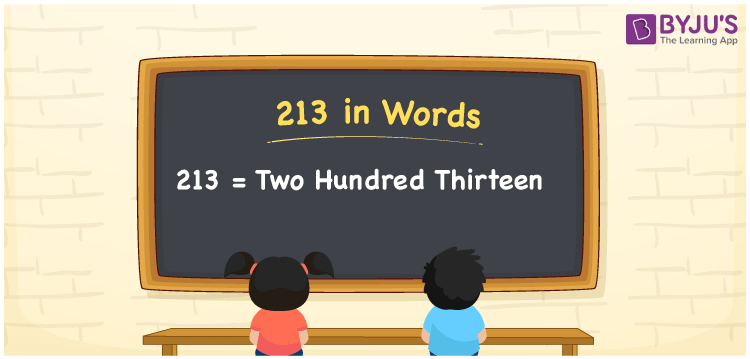# 213 in Words

213 in words is written as Two hundred thirteen. In both the International System of Numerals and the Indian System of Numerals, 213 is written as Two hundred thirteen. The number 213 is a Cardinal Number as it represents some quantity. For example, “there are 213 people in the room”.

 213 in Words Two hundred thirteen Two hundred thirteen in Number 213

## 213 in English Words

We write 213 in English Words using the letters of the English alphabet. Therefore, we read 213 in English as “Two hundred thirteen.”## How to Write 213 in Words?

To write 213 in words, we shall use the place value chart. In the place value chart, write 2 in the hundreds, 1 in the tens, and 3 in the ones, respectively. Now let us make a place value chart to write the number 213 in words.

 Hundreds Tens Ones 2 1 3

Thus, we can write the expanded form as

2 × Hundred + 1 × Ten + 3 × One

= 2 × 100 + 1 × 10 + 3 × 1

= 200 + 10 + 3

= 213

= Two hundred thirteen.

213 is a natural number, the successor of 212 and the predecessor of 214.

213 in words – Two hundred thirteen

• Is 213 an odd number? – Yes
• Is 213 an even number? – No
• Is 213 a perfect square number? – No
• Is 213 a perfect cube number? – No
• Is 213 a prime number? – No
• Is 213 a composite number? – Yes

## Frequently Asked Questions on 213 in Words

Q1

### How to write 213 in words?

213 in words is written as Two hundred thirteen.
Q2

### How to write 213 in the International and Indian System of Numerals?

In both the system of numerals, 213 in words, is written as Two hundred thirteen.
Q3

### What are the factors of 213?

The factors of 213 are 1, 3, 71 and 213.